Question

(I) A novice skier, starting from rest, slides down an icy frictionless 8.0° incline whose vertical height is 105 m. How fast is she going when she reaches the bottom?

1.niczorrrr

v = 45.37 m/s

Explanation:

Given,

angle of inclination = 8.0°

Vertical height, H  = 105 m

Initial K.E. = 0 J

Initial P.E. = m g H

Final PE = 0 J

Final KE =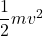Using Conservation of energy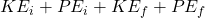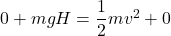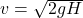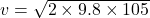v = 45.37 m/s

Hence, speed of the skier at the bottom is equal to v = 45.37 m/s